# 7 Derivative Rules You Should Know

## Important concepts and formulas for the basis of differential calculus

If you’ve taken any sort of calculus course, you will have undoubtedly been introduced to the derivative. It is one of the most important aspects of mathematics, and it serves as the basis for all topics in calculus.

We define the derivative as the rate of change of a function with respect to a variable, usually x. However, as always, mathematicians have come to represent this definition in a much more math-y way, which is called the limit definition.

You’ll notice that the expression next to the limit is essentially an expression of slope. We’re starting at some point x on a function and calculating the slope of the line created by another point on the function h units away. But, we take the limit of this slope as h approaches 0, or as the distance between the two x-coordinates of the line shrinks to 0; we eventually get the tangent line of the function at the point x, which is our derivative. Now that we have covered the basics of the derivative, let’s dive into our rules.

# Rule #1: Constant Function Rule

If f is a function that is a constant c, then the derivative of f is zero.

Intuitively, this makes sense because the slope of a constant line at any point is always 0: there is no rate of change. Therefore, if you are given y = 5, y = -5 or y = 1,000,000, the derivative of all of those functions is always the same: ZERO.

# Rule #2: Constant Multiple Rule

The derivative of a constant multiplied by a function is equal to the constant itself multiplied by the derivative of the function.

Note here that c represents the constant that we are talking about

For example, let’s say we wanted to find the derivative of 4x³. We recognize that there is a constant 4, so we can apply the constant multiple rule. All that needs to be done is to find the derivative of x³ and multiply it by 4. Doing so yields 4(3x²) = 12x². In the next rule, we’ll understand how we evaluated the derivative of x³.

# Rule #3: Power Rule

The derivative of x raised to a power is the value of the power times x raised to the quantity of the power minus one.

Now, we can see how we computed the derivative of x³ from the previous example. The power involved is 3, so we bring that down as the constant and multiply it by x².

# Rule #4: Sum Rule

The derivative of a sum is the sum of the derivatives.

This is often used to break down problems that look more complex on the surface. Let’s take the expression x³ + 3x⁵. Without the Sum Rule, we may have some trouble when trying to differentiate. However, we can just differentiate the individual terms here and add the results. By the Power Rule, we know that the derivative of x³ is 3x², and the derivative of 3x⁵ is 15x⁴. Therefore, the derivative of the original expression is 3x² + 15x⁴. What’s neat is that no matter how many terms there are, as long as they are summed together, this rule is applicable.

Note that this rule also applies to differences. In that case, the rule becomes the derivative of a difference is the difference of the derivatives.

# Rule #5: Product Rule

The derivative of a product of two functions is the sum of the first function multiplied by the derivative of the second function and the derivative of the first function multiplied by the second function.

Now we are getting into the more interesting and complicated rules. Let’s say that we wanted to compute the derivative of the expression (x + 1)(x² + 1). By the Product Rule, the first part of our answer would be (x + 1)(2x), and the second part would be (x² + 1)(1). Our final answer is then, 3x² + 2x + 1.

# Rule #6: Quotient Rule

The derivative of the quotient of two functions is the difference of the function in the denominator multiplied by the derivative of the function in the numerator and the function in the numerator multiplied by the derivative of the function in the denominator, all divided by the function in the denominator squared (this may be confusing, so refer at the equation below).

This rule may be the most complicated out of the bunch. Unfortunately, there are no tricks involved here, and the process of using the quotient rule is about as boring as it looks. For example, let’s say we wanted to compute the derivative of the expression (x + 1) / (x - 1). The numerator will end up being (x - 1)(1) - (x + 1)(1), or -2, and the denominator will end up being (x - 1)². Therefore, our final answer is -2 / (x - 1)².

# Rule #7: Chain Rule

The derivative of a composition of two functions is the derivative of the “outer function” with the input of the “inside function” multiplied by the derivative of the “inside function.”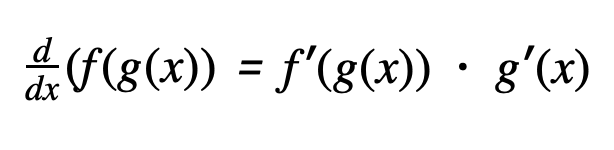Screenshot from author. Note that for simplicity, I’ve used ‘ to represent the action of taking the derivative.

The chain rule is often confusing, and it can cause some problems if it isn’t properly recognized. For example, take the task of differentiating sin(2x). Many people would’ve written cos(2x), circled it, and called it a day. However, we must realize that the input of 2x is its own function! Therefore, we need to take its derivative and multiply it by cos(2x). The final solution is then 2cos(2x).

I hope you enjoyed this overview of the basic derivative rules. It is important to grasp these concepts earlier rather than later, as they will show up a lot in more complicated mathematics courses. Trust me, your life will become much easier if you’ve got these down cold!

--

--

--

## More from Albert Ming

18 || High School Senior || Stats & Math

Love podcasts or audiobooks? Learn on the go with our new app.

## Number Fields## Rotating a graph around the y-axis - How would we see it on an x-y plane?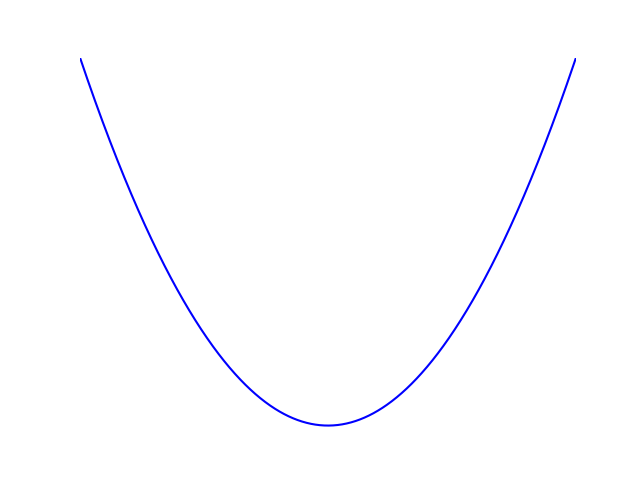## Multiplication Tables is the foundation for passing Maths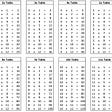## Quantum Phase Estimation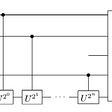## How to Implement Normal Distribution in DAX — DAX in Power BI — Chapter 14## Black-Scholes Derivation — Portfolio Replication Argument## The Powers of Two: An Introduction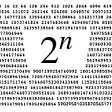## Solving a Polar Coordinate Problem in Calculus.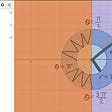## Albert Ming

18 || High School Senior || Stats & Math

## Wait, Change is Better Than Quantity?## 1. Math Basics and Linear Algebra## Embed math equations in your articles.## A Closer Look at the Derivative